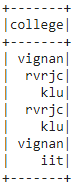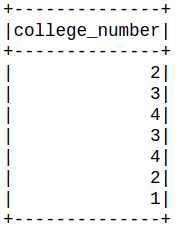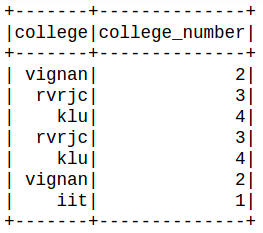# Pyspark Dataframe – Map Strings to Numeric

• Last Updated : 24 Sep, 2021

In this article, we are going to see how to convert map strings to numeric.

#### Creating dataframe for demonestration:

Here we are creating a row of data for college names and then pass the createdataframe() method and then we are displaying the dataframe.

Attention geek! Strengthen your foundations with the Python Programming Foundation Course and learn the basics.

To begin with, your interview preparations Enhance your Data Structures concepts with the Python DS Course. And to begin with your Machine Learning Journey, join the Machine Learning - Basic Level Course

## Python3

 `# importing module``import` `pyspark` `# importing sparksession from pyspark.sql module and Row module``from` `pyspark.sql ``import` `SparkSession,Row` `# creating sparksession and giving an app name``spark ``=` `SparkSession.builder.appName(``'sparkdf'``).getOrCreate()` `# list  of college data``dataframe ``=` `spark.createDataFrame([Row(``"vignan"``),``                                   ``Row(``"rvrjc"``),``                                   ``Row(``"klu"``),``                                   ``Row(``"rvrjc"``),``                                   ``Row(``"klu"``),``                                   ``Row(``"vignan"``),``                                   ``Row(``"iit"``)],``                                  ``[``"college"``])` `# display dataframe``dataframe.show()`

Output:## Method 1: Using map() function

Here we created a function to convert string to numeric through a lambda expression

Syntax: dataframe.select(“string_column_name”).rdd.map(lambda x: string_to_numeric(x)).map(lambda x: Row(x)).toDF([“numeric_column_name”]).show()

where,

• dataframe is the pyspark dataframe
• string_column_name is the actual column to be mapped to numeric_column_name
• string_to_numericis the function used to take numeric data
• lambda expression is to call the function such that numeric value is returned

Here we are going to create a college spark dataframe using the Row method and then we are going to map the numeric value by using the lambda function and rename college name as college_number. For that, we are going to create a function and check the condition and return numeric value 1 if college is IIT, return numeric value 2 if college is vignan, return numeric value 3 if college is rvrjc, return numeric value 4 if college is other than above three

## Python3

 `# function that converts string to numeric``def` `string_to_numeric(x):``  ` `      ``# return numeric value 1 if college is iit``    ``if``(x ``=``=` `'iit'``):``       ``return` `1``    ``elif``(x ``=``=` `"vignan"``):``      ` `    ``# return numeric value 2 if college is vignan``       ``return` `2``    ``elif``(x ``=``=` `"rvrjc"``):``  ` `      ``# return numeric value 3 if college is rvrjc``       ``return` `3``    ``else``:``      ` `    ``# return numeric value 4 if college``    ``# is other than above three``       ``return` `4` `# map the  numeric value by using lambda``# function and rename college name as college_number``dataframe.select(``"college"``).``rdd.``map``(``lambda` `x: string_to_numeric(x[``0``])).``map``(``lambda` `x: Row(x)).toDF([``"college_number"``]).show()`

Output:## Method 2: Using withColumn() method.

Here we are using withColumn() method to select the columns.

Syntax: dataframe.withColumn(“string_column”, when(col(“column”)==’value’, 1)).otherwise(value))

Where

• dataframe is the pyspark dataframe
• string_column is the column to be mapped to numeric
• value is the numeric value

Example: Here we are going to create a college spark dataframe using Row method and  map college name with college number using with column method along with when().

## Python3

 `# import col and when modules``from` `pyspark.sql.functions ``import` `col, when` `# map college name with college number``# using with column method along with when module``dataframe.withColumn(``"college_number"``,``                     ``when(col(``"college"``)``=``=``'iit'``, ``1``)``                     ``.when(col(``"college"``)``=``=``'vignan'``, ``2``)``                     ``.when(col(``"college"``)``=``=``'rvrjc'``, ``3``)``                     ``.otherwise(``4``)).show()`

Output:My Personal Notes arrow_drop_up